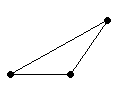# Triangles: Classification

A triangle is a polygon with three sides.

Triangles can be classified by side length or by angle measure .

 Classification by Side Length Equilateral Triangle All sides congruent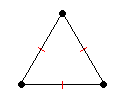Isosceles Triangle At least two sides congruent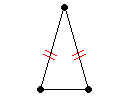Scalene Triangle No sides congruent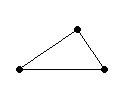Classification by Angle Measure Acute Triangle All interior angles measure less than $90°$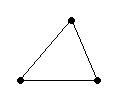Right Triangle Exactly one interior angle measures $90°$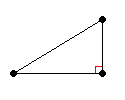Obtuse Triangle Exactly one interior angle measures more than $90°$